Courses

# Complex Number MCQ Level - 1

## 10 Questions MCQ Test Topic wise Tests for IIT JAM Physics | Complex Number MCQ Level - 1

Description
This mock test of Complex Number MCQ Level - 1 for IIT JAM helps you for every IIT JAM entrance exam. This contains 10 Multiple Choice Questions for IIT JAM Complex Number MCQ Level - 1 (mcq) to study with solutions a complete question bank. The solved questions answers in this Complex Number MCQ Level - 1 quiz give you a good mix of easy questions and tough questions. IIT JAM students definitely take this Complex Number MCQ Level - 1 exercise for a better result in the exam. You can find other Complex Number MCQ Level - 1 extra questions, long questions & short questions for IIT JAM on EduRev as well by searching above.
QUESTION: 1

###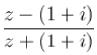is purely imaginary then z lies on :

Solution: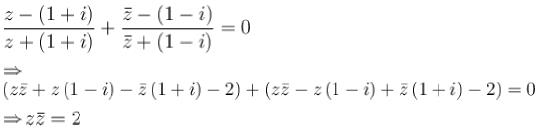locus of z is circle.
The correct answer is: a circle

QUESTION: 2

### Vector z = 3 - 4i is turned anticlockwise throuah an anale 180° and stretched 2.5 times. Complex number corresponding to newly obtained vector is :

Solution:

z = 3 - 4i
Newly obtained vector is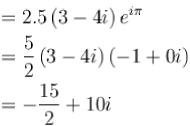The correct answer is: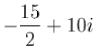QUESTION: 3

### If 1, α1, α2,..................αn-1 are nth root of unity then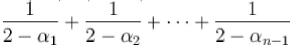is :

Solution:

( xn - 1) = ( x - 1 ) ( x - α1) (x - α2)......(x - α- 1)
log(xn - 1) = log (x - 1) + log ( x - α1) +.......+ log ( x - αn - 1)
Differentiate w.r.t. x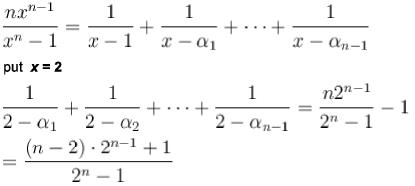The correct answer is: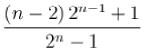QUESTION: 4

If z = cos θ + i sin θ, then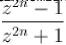is equal to :

Solution: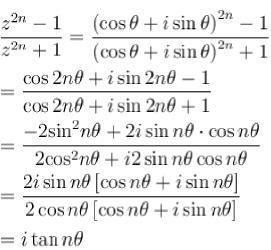The correct answer is: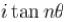QUESTION: 5

If conjugate of ( x + iy) ( 1 - 2i ) be 1 + i the x and y are :

Solution: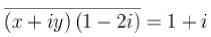⇒ ( x - iy)(1 + 2i) = 1 + i
so x + 2y = 1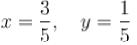The correct answer is: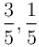QUESTION: 6

Amplitude of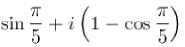Solution: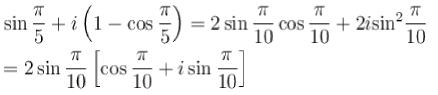so amplitude =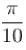The correct answer is:QUESTION: 7

If  ω≠ 1 is a cube root of unity, then sum of series
S = 1 + 2ω + 3ω + .... + 3nω3n-1(n ∈ N )

Solution: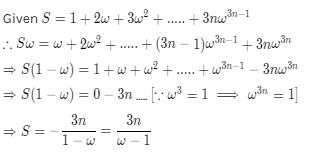QUESTION: 8

If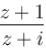is purely imaginary, then z lies on a :

Solution: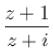is purely imaginary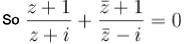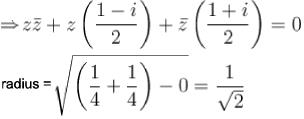QUESTION: 9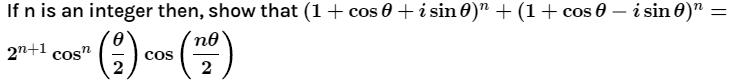Solution: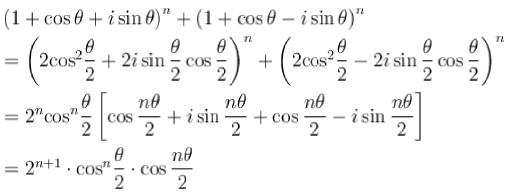The correct answer is: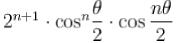QUESTION: 10

If (x - iy) + i (3x + iy) =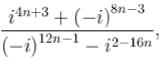n ∈ N then pair (x,y) is :

Solution: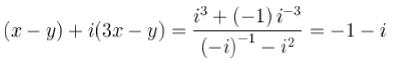by comparison x - y = - 1
x - y = -1
3x - y = -1
So, x = 0, y = 1